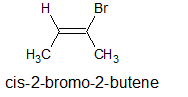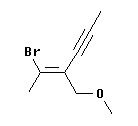# 7.5: The E/Z System (when cis/trans does not work)

Learning Objective

• recognize and classify the stereochemistry of alkenes using the cis/trans and E/Z systems
• give the IUPAC names for alkenes given their structure & vice versa including E/Z isomers

## E/Z Nomenclature in Alkenes

The traditional system for naming the geometric isomers of an alkene, in which the same groups are arranged differently, is to name them as cis or trans. However, it is easy to find examples where the cis-trans system is not easily applied. IUPAC has a more complete system for naming alkene isomers. The R-S system for chirality is based on a set of "priority rules", which allow you to rank any groups. The rigorous IUPAC system for naming alkene isomers, called the E-Z system, is based on the same priority rules.

The priority rules are often called the Cahn-Ingold-Prelog (CIP) rules, after the chemists who developed the system.

The general strategy of the E-Z system is to analyze the two groups at each end of the double bond. For each vinyl carbon, rank the two groups using the CIP priority rules. Determine whether the higher priority group at one end of the double bond and the higher priority group at the other end of the double bond are on the same side (Z, from German zusammen = together or "on Zee Zame Zide") or on opposite sides (E, from German entgegen = opposite) of the double bond.

Example $$\PageIndex{1}$$

The figure below shows the two isomers of 2-butene. You should recognize them as cis and trans. Let's analyze them to see whether they are E or Z. Start with the left hand structure (the cis isomer). On C2 (the left end of the double bond), the two atoms attached to the double bond are C and H. By the CIP priority rules, C is higher priority than H (higher atomic number). Now look at C3 (the right end of the double bond). Similarly, the atoms are C and H, with C being higher priority. We see that the higher priority group is "down" at C2 and "down" at C3. Since the two priority groups are both on the same side of the double bond ("down", in this case), they are zusammen = together. Therefore, this is (Z)-2-butene.

Now look at the right hand structure (the trans isomer). In this case, the priority group is "down" on the left end of the double bond and "up" on the right end of the double bond. Since the two priority groups are on opposite sides of the double bond, they are entgegen = opposite. Therefore, this is (E)-2-butene.## E/Z will work -- even when cis/trans fails

In simple cases, such as 2-butene, Z corresponds to cis and E to trans. However, that is not a rule. This section and the following one illustrate some idiosyncrasies that happen when you try to compare the two systems. The real advantage of the E-Z system is that it will always work. In contrast, the cis-trans system breaks down with many ambiguous cases.

Example $$\PageIndex{1}$$

The following figure shows two isomers of an alkene with four different groups on the double bond, 1-bromo-2-chloro-2-fluoro-1-iodoethene.It should be apparent that the two structures shown are distinct chemicals. However, it is impossible to name them as cis or trans. On the other hand, the E-Z system works fine... Consider the left hand structure. On C1 (the left end of the double bond), the two atoms attached to the double bond are Br and I. By the CIP priority rules, I is higher priority than Br (higher atomic number). Now look at C2. The atoms are Cl and F, with Cl being higher priority. We see that the higher priority group is "down" at C1 and "down" at C2. Since the two priority groups are both on the same side of the double bond ("down", in this case), they are zusammen = together. Therefore, this is the (Z) isomer. Similarly, the right hand structure is (E).

## E/Z will work, but may not agree with cis/trans

There are also molecules for which the E/Z system will not agree with the cis/trans system. Let's use 2-bromo-2-butene to explore this option. Is this compound cis or trans? This molecule is clearly cis. The two methyl groups are on the same side. More rigorously, the "parent chain" is cis.Is this compound E or Z? There is a methyl at each end of the double bond. On the left, the methyl is the high priority group because the other group is -H. On the right, the methyl is the low priority group because the other group is -Br. That is, the high priority groups are -CH3 (left) and -Br (right). Thus the two priority groups are on opposite sides = entgegen = E.This example should convince you that cis and Z are not synonyms. Cis/trans and E,Z are determined by distinct criteria. There may seem to be a simple correspondence, but it is not a rule. Be sure to determine cis,trans or E,Z separately, as needed.

## Multiple double bonds

If the compound contains more than one double bond, then each one is analyzed and declared to be E or Z.

Example $$\PageIndex{3}$$The configuration at the left hand double bond is E; at the right hand double bond it is Z. Thus this compound is (1E,4Z)-1,5-dichloro-1,4-hexadiene.

## The double-bond rule in determining priorities

Example $$\PageIndex{4}$$

Consider the compound belowThis is 1-chloro-2-ethyl-1,3-butadiene -- ignoring, for the moment, the geometric isomerism. There is no geometric isomerism at the second double bond, at 3-4, because it has 2 H at its far end.

What about the first double bond, at 1-2? On the left hand end, there is H and Cl; Cl is higher priority (by atomic number). On the right hand end, there is -CH2-CH3 (an ethyl group) and -CH=CH2 (a vinyl or ethenyl group). Both of these groups have C as the first atom, so we have a tie so far and must look further. What is attached to this first C? For the ethyl group, the first C is attached to C, H, and H. For the ethenyl group, the first C is attached to a C twice, so we count it twice; therefore that C is attached to C, C, H. CCH is higher than CHH; therefore, the ethenyl group is higher priority. Since the priority groups, Cl and ethenyl, are on the same side of the double bond, this is the Z-isomer; the compound is (Z)-1-chloro-2-ethyl-1,3-butadiene.

## The "first point of difference" rule

Which is higher priority, by the CIP rules: a C with an O and 2 H attached to it or a C with three C? The first C has one atom of high priority but also two atoms of low priority. How do these "balance out"? Answering this requires a clear understanding of how the ranking is done. The simple answer is that the first point of difference is what matters; the O wins.To illustrate this, consider the molecule at the left. Is the double bond here E or Z? At the left end of the double bond, Br > H. But the right end of the double bond requires a careful analysis.

At the right hand end, the first atom attached to the double bond is a C at each position. A tie, so we look at what is attached to this first C. For the upper C, it is CCC (since the triple bond counts three times). For the lower C, it is OHH -- listed in order from high priority atom to low. OHH is higher priority than CCC, because of the first atom in the list. That is, the O of the lower group beats the C of the upper group. In other words, the O is the highest priority atom of any in this comparison; thus the O "wins".

Therefore, the high priority groups are "up" on the left end (the -Br) and "down" on the right end (the -CH2-O-CH3). This means that the isomer shown is opposite = entgegen = E. And what is the name? The "name" feature of ChemSketch says it is (2E)-2-(1-bromoethylidene)pent-3-ynyl methyl ether.

Example $$\PageIndex{1}$$

The configuration about double bonds is undoubtedly best specified by the cis-trans notation when there is no ambiguity involved. Unfortunately, many compounds cannot be described adequately by the cis-trans system. Consider, for example, configurational isomers of 1 -fluoro- 1 -chloro-2- bromo-2-iodo-ethene, 9 and 10. There is no obvious way in which the cis-trans system can
be used:A system that is easy to use and which is based on the sequence rules already described for the R,S system works as follows:

1. An order of precedence is established for the two atoms or groups attached to each end of the double bond according to the sequence rules of Section 19-6. When these rules are applied to 1-fluoro- 1-chloro-2-bromo-2- iodoethene, the priority sequence is:
• at carbon atom 1, C1 > F
• at carbon atom 2, I > Br
1. Examination of the two configurations shows that the two priority groups- one on each end- are either on the same side of the double bond or on opposite sides:The Z isomer is designated as the isomer in which the top priority groups are on the same side (Z is taken from the German word zusammen- together). The E isomer has these groups on opposite sides (E, German for entgegen across). Two further examples show how the nomenclature is used:## Exercises

1. Order the following in increasing priority.

A) –H, –Cl, –OH

B) –CH3, CH2OH, CH2CH3

C) –C≡CH, –CH=CH2, –CH=O

2. Label the following as E or Z conformations.1. A) –H < OH < Cl (highest priority)

B) –CH3 < CH2CH3 < CH2OH (highest priority)

C) –CH=CH2 < C≡CH < CH=O (highest priority)

2. A) Z B) Z C) E